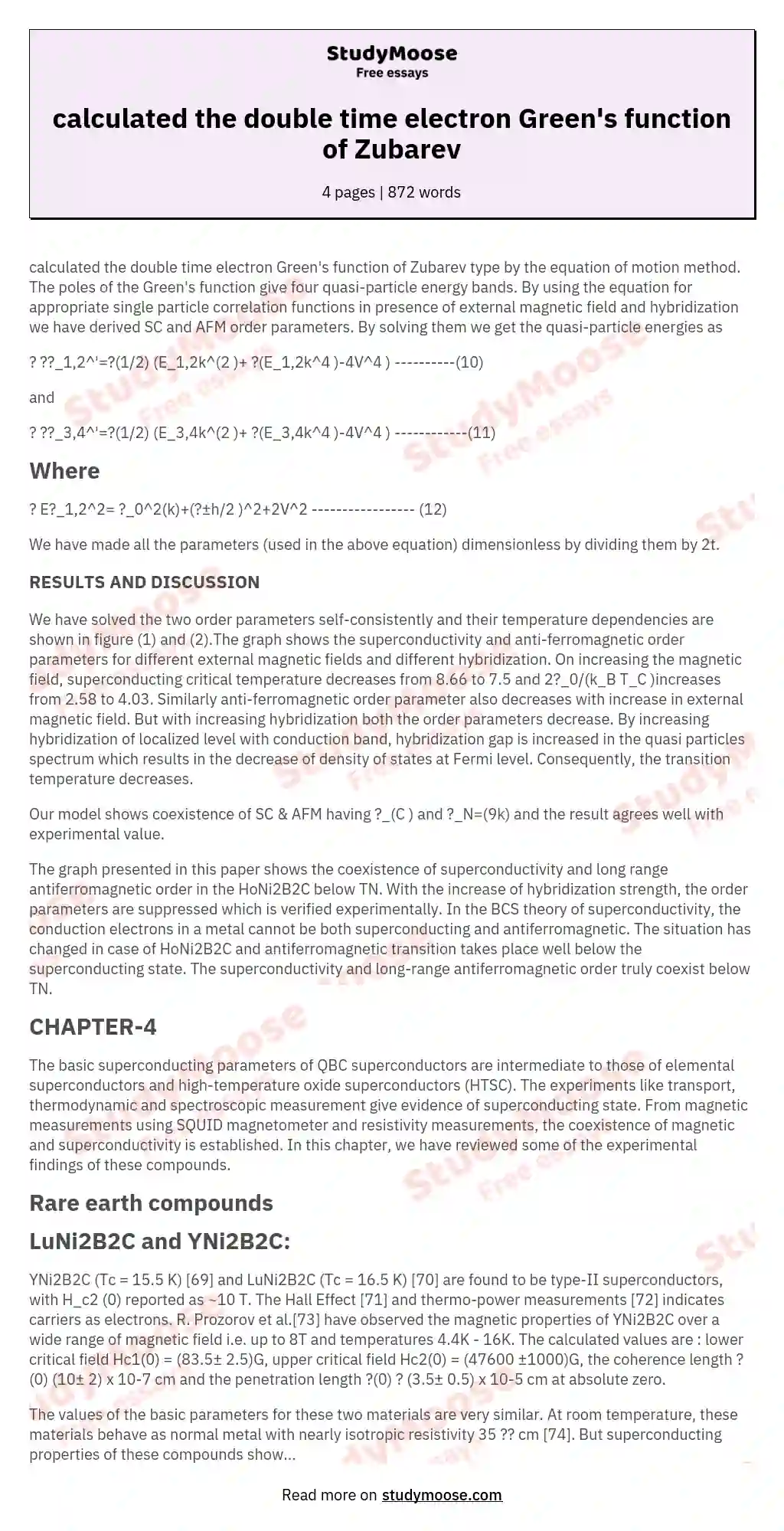# calculated the double time electron Green's function of Zubarev

Categories: Time

calculated the double time electron Green’s function of Zubarev type by the equation of motion method. The poles of the Green’s function give four quasi-particle energy bands. By using the equation for appropriate single particle correlation functions in presence of external magnetic field and hybridization we have derived SC and AFM order parameters. By solving them we get the quasi-particle energies as

? ??_1,2^’=?(1/2) (E_1,2k^(2 )+ ?(E_1,2k^4 )-4V^4 ) ———-(10)

and

? ??_3,4^’=?(1/2) (E_3,4k^(2 )+ ?(E_3,4k^4 )-4V^4 ) ————(11)

## Where

? E?_1,2^2= ?_0^2(k)+(?±h/2 )^2+2V^2 —————– (12)

We have made all the parameters (used in the above equation) dimensionless by dividing them by 2t.

### RESULTS AND DISCUSSION

We have solved the two order parameters self-consistently and their temperature dependencies are shown in figure (1) and (2).The graph shows the superconductivity and anti-ferromagnetic order parameters for different external magnetic fields and different hybridization. On increasing the magnetic field, superconducting critical temperature decreases from 8.66 to 7.5 and 2?_0/(k_B T_C )increases from 2.58 to 4.03. Similarly anti-ferromagnetic order parameter also decreases with increase in external magnetic field.

Get quality help nowKarrieWritesVerified writer

Proficient in: Time5 (339)

“ KarrieWrites did such a phenomenal job on this assignment! He completed it prior to its deadline and was thorough and informative. ”+84 relevant experts are online

But with increasing hybridization both the order parameters decrease. By increasing hybridization of localized level with conduction band, hybridization gap is increased in the quasi particles spectrum which results in the decrease of density of states at Fermi level. Consequently, the transition temperature decreases.

Our model shows coexistence of SC & AFM having ?_(C ) and ?_N=(9k) and the result agrees well with experimental value.

The graph presented in this paper shows the coexistence of superconductivity and long range antiferromagnetic order in the HoNi2B2C below TN.

Get to Know The Price Estimate For Your Paper
Topic
Number of pages
Email Invalid email

You won’t be charged yet!

With the increase of hybridization strength, the order parameters are suppressed which is verified experimentally. In the BCS theory of superconductivity, the conduction electrons in a metal cannot be both superconducting and antiferromagnetic. The situation has changed in case of HoNi2B2C and antiferromagnetic transition takes place well below the superconducting state. The superconductivity and long-range antiferromagnetic order truly coexist below TN.

## CHAPTER-4

The basic superconducting parameters of QBC superconductors are intermediate to those of elemental superconductors and high-temperature oxide superconductors (HTSC). The experiments like transport, thermodynamic and spectroscopic measurement give evidence of superconducting state. From magnetic measurements using SQUID magnetometer and resistivity measurements, the coexistence of magnetic and superconductivity is established. In this chapter, we have reviewed some of the experimental findings of these compounds.

## LuNi2B2C and YNi2B2C:

YNi2B2C (Tc = 15.5 K)  and LuNi2B2C (Tc = 16.5 K)  are found to be type-II superconductors, with H_c2 (0) reported as ~10 T. The Hall Effect  and thermo-power measurements  indicates carriers as electrons. R. Prozorov et al. have observed the magnetic properties of YNi2B2C over a wide range of magnetic field i.e. up to 8T and temperatures 4.4K – 16K. The calculated values are : lower critical field Hc1(0) = (83.5± 2.5)G, upper critical field Hc2(0) = (47600 ±1000)G, the coherence length ? (0) (10± 2) x 10-7 cm and the penetration length ?(0) ? (3.5± 0.5) x 10-5 cm at absolute zero.

The values of the basic parameters for these two materials are very similar. At room temperature, these materials behave as normal metal with nearly isotropic resistivity 35 ?? cm . But superconducting properties of these compounds show anisotropy which raises the question of symmetry of order parameter. Similarly the penetration depth, ?(0) is ~1000 ? and coherence length ?(0) is ~100 ?. The ratio, ?C/?Tc for YNi_2 B_2 C is 1.8 compared to 1.43 as expected from BCS theory. Here ?C is the jump in heat capacity at Tc and ? is the coefficient of electron specific heat. The superconducting energy gap as measured by different experiments like tunneling, point contact Andreev reflection spectroscopy [78-80] for the above two compounds are ? (0) ~2.2 meV with ? /k_B T_c ~ 2 against the value of 1.76 as in BCS theory gap energy.

All these facts show that these materials are conventional intermediate to strong coupling superconductors. Isotope effect in YNi2B2C and LuNi2B2C [75, 76] indicate that conventional electron-phonon interaction as the mechanism for superconductivity. In YNi2B2C, the partial exponents for the 10B and 11B isotopes have been found to be 0.21 and 0.11, respectively , as against about 0.4 expected theoretically. This shows that other elements in the system may also be contributing to the cause of superconductivity. Mossbauer resonance of superconducting DyNi2B2C is similar to that of a non-metallic system, which indirectly suggests that superconductivity essentially arises from Ni_2 B_2layer .

P. Raychaudhuri et.al.  have studied the anisotropy in the superconducting energy gap in a single crystal of YNi2B2C (Tc~ 14:6 K) using directional point-contact spectroscopy as shown in Fig-1.

C. L. Huang et.al.  have measured the magnetic field dependence of low-temperature specific heat in YNi2B2C using various pairing order parameters. They have obtained two energy gaps, ?L=2.67meV, ?S=1.19 meV and nonlinear field dependence of electronic specific heat coefficient, ?(H) ~H0.47, which is quantitatively close to ?(H) ~H0.5 (Fig-3). This isexpected for nodal superconductivity or can be qualitatively explained using two-gap scenario. Furthermore, the positive curvature in Hc2 (T) near Tc is qualitatively similar to that in the other two-gap superconductor MgB2.

## HoNi2B2C :

The coexistence of superconductivity magnetic order is obtained for HoNi2B2C with (Tc ~ 8 K and TN ~ 8 K) . From neutron diffraction method J.W. Lynn et al.  have obtained Tc~8K for superconducting HoNi2B2C and Tc

### Similar topics:

Physics Essay Sxamples Chemistry Essay Ideas For Research Paper